# Fraction Word Problems

#### Number of problems found: 627

• Milk cratesA farmer sells milk in crates that hold 15 bottles. She has 34,125 bottles of milk. How many crates can the farmer fill?After the history test, Michaella discovered that the ratio of her correct and incorrect answers is 5: 3. How many correct answers did Michaella have in the test, if she had 6 incorrect answers?
• Borrowing bicycle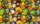Jakub lent a bicycle to friends who wanted to ride it. For a three-hour bike ride, Jakub received 2 chocolates. Yup. If you wanted a bike for 2 hours, you had to give Jakub 12 candies. Peter gave Jakub 1 chocolate and 3 candies. How long can Peter ride Ja
• A quotientWhat is the quotient of 3/10 divided by 2/4 as a fraction
• A bucketA bucket has 4 liters of water in it when it is 2/5 full. How much can it hold?7 is added to the sum of 4/5 and 6/7
• Pizza palaceJosh is at Enzo's pizza palace. He can sit at a table with 5 of his friends or at a different table with seven of his friends. The same size pizza is shared equally among the people at each table. At which table should Josh sit to get more pizza? (write a
• An electricianAn electrician needs 1 1/3 rolls of electrical wire to wire each room in a house. How many rooms can he wire with 6 2/3 rolls of wire?
• Divide in ratioLine segment AB 12 cm long divide in a ratio of 5: 3. How long are the individual parts?
• From aFrom a 1 meter ribbon, Ericka cut 2/4 meter for her hat and another 1/4 meter for her bag. How long was the remaining piece?
• LibraryNew books were purchased for the library. Five-eighths were professional books, one-fifth were encyclopedias, and 231 books were dictionaries. How many professional books were there?
• Spending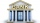Peter spends 1/5 of his earnings on his rent and he saves 2/7. What fraction of his earnings is left?
• The tourist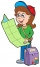The tourist walked a quarter of the way on the first day, a third of the rest on the second day, and 20 km on the last day. How many km did he walk in three days?
• Simplest form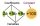Find the simplest form of the following expression: 3 to the 2nd power - 1/4 to the 2nd power
• Drawing from a hatWhen drawing numbers from a hat from 1 to 35, we select random given numbers. What is the probability that the drawn numbers will be divisible by 8 and 2?
• Job applicantsJob applicants: three-fourths of applicants had experience for a position. The number that did not have prior experience was 36. How many people applied for the job?
• GrandmaWe're going to Grandma. We still have 24 km left. How far does Grandma live if we have traveled from the total distance: half, a third, a fifth?
• Iron pole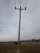The iron pole is in the ground 2/5 of its length, partly above the ground 1/3 is yellow, and the unpainted section is 6 m long. How long is the entire column?
• Ducats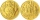The king divided the ducats to his three sons in a ratio of 2: 5: 4. How many ducats the king divided them if the youngest received 260 ducats, which was the least of all sons.
• Tourist routeHow long is the tourist route when tourists crossed four-sevenths of the way on foot, crossed the bus twice less than on foot and passed the last 14 kilometers by boat.

Do you have an interesting mathematical word problem that you can't solve it? Submit a math problem, and we can try to solve it.

We will send a solution to your e-mail address. Solved examples are also published here. Please enter the e-mail correctly and check whether you don't have a full mailbox.

Please do not submit problems from current active competitions such as Mathematical Olympiad, correspondence seminars etc...

Need help calculate sum, simplify or multiply fractions? Try our fraction calculator.